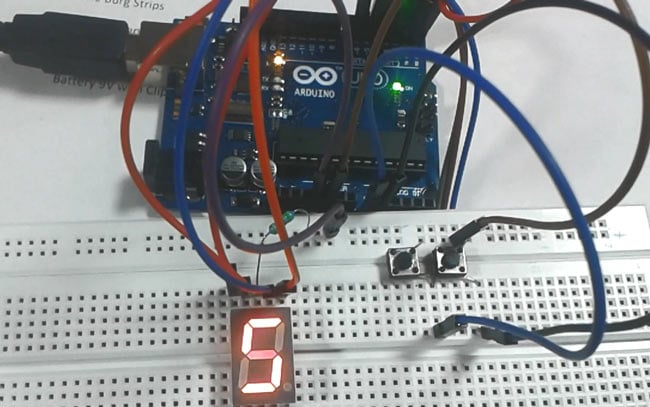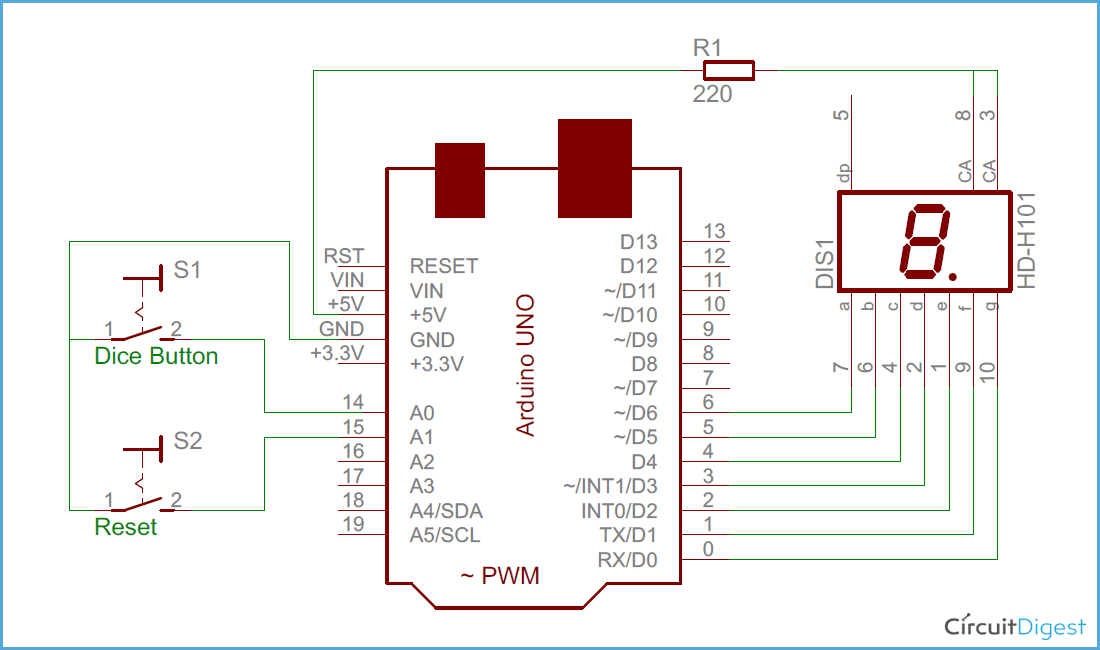# Digital Dice using Arduino

Published  July 2, 2015   3
S
AuthorWe all are familiar with dice and often played LUDO or SANP SIDI (Snake & Ladders) game by using dice. Dice is a squire type solid box which contains 6 different numbers on all of its sides. We throw dice on a surface to get a random number while playing the games. In this project we have tried to replicate it with a digital dice using arduino uno board. In place of throwing the dice, here we need to press a button to get a random number between 0 to 6.

### Required Components

1. Arduino UNO
2. Seven segment display (Common Anode)
3. Push buttons
4. Connecting wires
6. 1 k resistor
7. Power supply

### Circuit Diagram and ExplanationAs shown in the above digital dice circuit, arduino is used for controlling whole the process. Two push buttons are used in the circuit - one to start the dice and other for resetting the dice. Arduino reads these two buttons and perform the operation. A seven segment display is used to display the dice result.

Arduino is continuously executing rand() function and stored its value in to a temporary variable. When dice button is get pressed stored value calculated and display on seven segment by using appropriate method (see programming part of article).

Here in this dice circuit, a common anode seven segment display is used for displaying dice numbers, which is directly connected to arduino digital pin numbers 6, 5, 4, 3, 2, 1, 0. And common anode pin of seven segment is connected with +5 volt 220 Ohm resistor. Two push button are also connected namely dice button and reset button which are connected to digital pin 14 (A0) and 15 (A1) with respect to ground.

### Code Explanation

Programming part of this project plays a very important role to display random digital dice number on seven segment display. Arduino does not contain any library for seven segment display. so we created whole code without using any library.

First of all we selects digital pin for seven segment display.

`int pin={6,5,4,3,2,1,0};`

After it we create an array of 6 digits of dice namely 1, 2, 3, 4, 5 and 6.

`char digit={0x02, 0x79, 0x24, 0x30, 0x19, 0x12};`

Now we gave direction to use arduino pin as output.

```void setup()
{
for(int i=0;i<7;i++)
pinMode(pin[i], OUTPUT);
pinMode(dice, INPUT);
pinMode(resett, INPUT);
digitalWrite(dice, HIGH);
digitalWrite(resett, HIGH);```

We send a code for displaying zero on seven segment display by default.

```int temp=0x40;
for(int i=0;i<7;i++)
{
int temp1=temp&0x01;
digitalWrite(pin[i], temp1);
temp=temp>>1;
}```

Now we run rand() function to get a random number.

`int temp=rand();`

And when we press the dice button program first map this random number and then send number to seven segment display by using bit wise operator.

```if(digitalRead(dice)==0)
{
int k=temp%6;
temp=digit[k];
wait();
for(int i=0;i<7;i++)
{
int temp1=temp&0x01;
digitalWrite(pin[i], temp1);
temp=temp>>1;
}
delay(200);
}```

And same for reset button.

In this program we sends single bit at a time. Here we applying a for loop which run 7 time to send data to each segment one by one.

Code

#define resett 15
#define dice 14

char digit={0x02, 0x79, 0x24, 0x30, 0x19, 0x12};
int pin={6,5,4,3,2,1,0};
void setup()
{
for(int i=0;i<7;i++)
pinMode(pin[i], OUTPUT);
pinMode(dice, INPUT);
pinMode(resett, INPUT);
digitalWrite(dice, HIGH);
digitalWrite(resett, HIGH);
int temp=0x40;
for(int i=0;i<7;i++)
{
int temp1=temp&0x01;
digitalWrite(pin[i], temp1);
temp=temp>>1;
}
delay(1000);
}

void loop()
{
int temp=rand();
{
int k=temp%6;
temp=digit[k];
wait();
for(int i=0;i<7;i++)
{
int temp1=temp&0x01;
digitalWrite(pin[i], temp1);
temp=temp>>1;
}
delay(200);
}

{
temp=0x40;
for(int i=0;i<7;i++)
{
int temp1=temp&0x01;
digitalWrite(pin[i], temp1);
temp=temp>>1;
}
}
}

void wait()
{
for(int m=0;m<10;m++)
{
for(int k=0;k<6;k++)
{
int ch=digit[k];
for(int l=0;l<7;l++)
{
char tem2=ch&0x01;
digitalWrite(pin[l], tem2);
ch=ch>>1;
}
delay(50);
}
}
}

Video

Tags

Submitted by Jorge on Fri, 11/20/2015 - 23:41

### Hey, nice project! My

Hey, nice project! My professor wants to me something like this, but with two displays. Does the reset button is necessary to the project or the dices can work without it:?

Submitted by Jorge on Sat, 11/21/2015 - 00:49

### Hey! Greetings from Brazil!

Hey! Greetings from Brazil! Can I do that withou a rest button? And how can I do a dice that choose numbers from 0 to 9?

Submitted by armandolindner on Tue, 06/12/2018 - 16:43